# Cohen-Macaulay ring

(diff) ← Older revision | Latest revision (diff) | Newer revision → (diff)

Macaulay ring

A commutative local Noetherian ring, the depth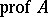of which is equal to its dimension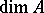. In homological terms, a Cohen–Macaulay ringis characterized as follows: The groups, or the local cohomology groups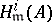, vanish for all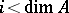, where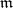is the maximal ideal inandis the residue field of. An alternative definition utilizes the concept of a regular sequence. A regular sequence is a sequence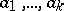of elements ofsuch that, for all, the element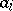is not a zero divisor in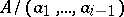. A local ringis a Cohen–Macaulay ring if there exists a regular sequencesuch that the quotient ring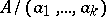is Artinian. In that case.

If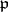is a prime ideal in a Cohen–Macaulay ring, then its height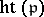(see Height of an ideal) satisfies the relationIn particular, a Cohen–Macaulay ring is equi-dimensional and it is a catenary ring. A fundamental result on Cohen–Macaulay rings is the following unmixedness theorem. Letbe a-dimensional Cohen–Macaulay ring anda sequence of elements ofsuch that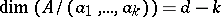. Thenis a regular sequence and the ideal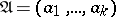is unmixed, i.e. any prime ideal associated withhas heightand co-height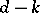. The unmixedness theorem was proved by F.S. Macaulay  for a polynomial ring and by I.S. Cohen  for a ring of formal power series.

Examples of Cohen–Macaulay rings. A regular local ring (and, in general, any Gorenstein ring) is a Cohen–Macaulay ring; any Artinian ring, any one-dimensional reduced ring, any two-dimensional normal ring — all these are Cohen–Macaulay rings. Ifis a local Cohen–Macaulay ring, then the same is true of its completion, of the ring of formal power series overand of any finite flat extension. A complete intersection of a Cohen–Macaulay ring, i.e. a quotient ring, whereis a regular sequence, is a Cohen–Macaulay ring. Finally, the localization of a Cohen–Macaulay ring in a prime ideal is again a Cohen–Macaulay ring. This makes it possible to extend the definition of a Cohen–Macaulay ring to arbitrary rings and schemes. Indeed, a Noetherian ring(a scheme) is called a Cohen–Macaulay ring (a Cohen–Macaulay scheme) if for any prime ideal(respectively, for any point) the local ring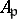(respectively,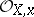) is a Cohen–Macaulay ring; for example, this is true of any semi-group ring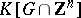, whereis a convex polyhedral cone in(see ).

Cohen–Macaulay rings are also stable under passage to rings of invariants. Ifis a finite group acting on a Cohen–Macaulay ring, and if moreover its order is invertible in, then the ring of invariantsis also a Cohen–Macaulay ring.

Ifis a graded ring, the property of being a Cohen–Macaulay ring appears in the cohomology of the invertible sheaves over the projective scheme(see ). If the homogeneous ringof a cone inassociated with a projective variety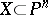is a Cohen–Macaulay ring, thenis called an arithmetical Cohen–Macaulay variety. In that case the ringis isomorphic to, and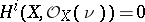for alland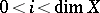, where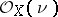is the-th tensor power of the polarized invertible sheaf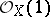on. This property holds for projective spaces and their products, complete intersections, Grassmann manifolds and Schubert subvarieties , flag manifolds and generalized flag manifolds .

A moduleover a local ringis called a Cohen–Macaulay module if its depth equals its dimension. Many results for Cohen–Macaulay rings carry over to Cohen–Macaulay modules; for example, the support of such a module is equi-dimensional. It has been conjectured that any local complete ringhas a Cohen–Macaulay modulesuch that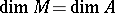.

How to Cite This Entry:
Cohen-Macaulay ring. Encyclopedia of Mathematics. URL: http://encyclopediaofmath.org/index.php?title=Cohen-Macaulay_ring&oldid=16394
This article was adapted from an original article by V.I. Danilov (originator), which appeared in Encyclopedia of Mathematics - ISBN 1402006098. See original article